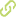# Distributed parameter system identification using finite element differential neural networks•
• Overview
•
• Identity
•
•
• View All
•

### Abstract

• © 2016 Elsevier B.V. All rights reserved.Most of the previous work on identification involves systems described by ordinary differential equations (ODEs). Many industrial processes and physical phenomena, however, should be modeled using partial differential equations (PDEs) which offer both spatial and temporal distributions that are simply not available with ODE models. Systems described by a PDE belong to a class of system called distributed parameter system (DPS). This article presents a method for solving the problem of identification of uncertain DPSs using a differential neural network (DNN). The DPS, assumed to be described by a PDE, is approximated using the finite element method (FEM). The FEM discretizes the domain into a set of distributed and connected nodes, thereby, allowing a representation of the DPS in a finite number of ODEs. The proposed DNN follows the same interconnection structure of the FEM, thus allowing the DNN to identify the FEM approximation of the DPS in both 2D and 3D domains. Lyapunov's second method was used to derive adaptive learning laws for the proposed DNN structure. The identification algorithm, here developed in Nvidia's CUDA/C to reduce the execution time, runs mostly on the graphics processing unit (GPU). A physical experiment served to validate the 2D case. In the experiment, the DNN followed the trajectory of 57 markers that were placed on an undulating square piece of silk. The proposed DNN is compared against a method based on principal component analysis and an artificial neural network trained with group search optimization. In addition to the 2D case, a simulation validated the 3D case, where input data for the DNN was generated by solving a PDE with appropriate initial and boundary conditions over an unitary domain. Results show that the proposed FEM-based DNN approximates the dynamic behavior of both a real 2D and a simulated 3D system.

• June 1, 2016